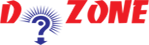﻿ Python program for converting Celsius to Fahrenheit## Python Program to Convert Celsius To Fahrenheit

Here in this tutorial, you will learn how to convert Celsius to Fahrenheit using python programming?

But to understand this, you must have the knowledge of some of the Python Programming topics:

• Data Types
• Input, Output and Import
• Operators

Here is the formula to run the Python Program:

``````
celsius * 1.8 = fahrenheit - 32
```
```
``````
Example:
# Python Program to convert temperature in celsius to fahrenheit

# change this value for a different result

celsius = 42.5

# calculate fahrenheit

fahrenheit = (celsius * 1.8) + 32

print('%0.1f degree Celsius is equal to %0.1f degree Fahrenheit' %(celsius,fahrenheit))

Output
42.5 degree Celsius is equal to 108.5 Degree Fahrenheit
```
```

Also, if you want to convert Fehrenheit into Celsius then again use the simple formula that is:

``````
celsius = (fahrenheit - 32) / 1.8
```
```

## Other Python Programs

PythonTraining, 258-FF , Katewa Nagar, New Sanganer Rd, Jaipur, 302019